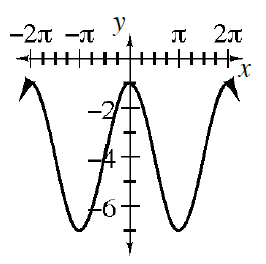### Home > CCA2 > Chapter 12 > Lesson 12.1.1 > Problem12-10

12-10.

Consider the function $f(x) = 3$ sin $(x+\frac { \pi } { 2 })-4$.

1. How is its graph different from $f(x)$ = sin$(x)$?

Look at the function's equation. What parts are different from the equation for $f(x)$ = sin$(x)$?
How is the graph shifted horizontally? Vertically? How is it stretched?

1. Sketch the graph.

Begin by drawing an axis that reflects the vertical shift. In this case it is $y = -4$.